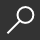## Introduction to Nonlinear Differential and Integral Equations by Harold T. Davis

Overview -

Within recent years interest in nonlinear equations has grown enormously. They are extremely important as basic equations in many areas of mathematical physics, and they have received renewed attention because of progress in their solution by machines.
This volume undertakes a definition of the field, indicating advances that have been made up through 1960. The author's position is that while the advent of machines has resulted in much new knowledge, one should not disregard analytical methods, since the solution of nonlinear equations possesses singularities which only the analytical method (as based upon the work of Poincare, Liapounoff, Painleve and Goursatl can discover.
After a general survey of the problem presented by nonlinear equations, the author discusses the differential equation of the first order, following this by chapters on the Riccati equation (as a bridge between linear and nonlinear equations) and existence theorems, with special reference to Cauchy's method. Second order equations are introduced via Volterra's problem and the problem of pursuit, and succeeding chapters cover elliptic integrals and functions and theta functions; differential equations of the second order; and second order differential equations of the polynomial class, with special reference to Painleve transcendents. The technique of continuous analytical continuation is shown, while phenomena of the phase plane are studied as an introduction to nonlinear mechanics. Nonlinear 111echanics is then discussed, with various classical equations like Van der Pol's equations, Emden's equation, and the Duffing problem. The remaining chapters are concerned with nonlinear integral equations, problems from the calculus of variations, and numerical integration of nonlinear equations. Throughout the book the results of distinguished analysis of the past and modern machine computations are both taken into account. Despite the thoroughness of its coverage this is a very fine introduction to this important area of mathematics, and it can easily be followed by the mathematically sophisticated reader who knows very little about nonlinear equations.

local_shippingFor DeliveryIn Stock.
FREE Shipping for Club Members helpNew & Used Marketplace 62 copies from \$3.45

## More About Introduction to Nonlinear Differential and Integral Equations by Harold T. Davis

### Overview

Within recent years interest in nonlinear equations has grown enormously. They are extremely important as basic equations in many areas of mathematical physics, and they have received renewed attention because of progress in their solution by machines.
This volume undertakes a definition of the field, indicating advances that have been made up through 1960. The author's position is that while the advent of machines has resulted in much new knowledge, one should not disregard analytical methods, since the solution of nonlinear equations possesses singularities which only the analytical method (as based upon the work of Poincare, Liapounoff, Painleve and Goursatl can discover.
After a general survey of the problem presented by nonlinear equations, the author discusses the differential equation of the first order, following this by chapters on the Riccati equation (as a bridge between linear and nonlinear equations) and existence theorems, with special reference to Cauchy's method. Second order equations are introduced via Volterra's problem and the problem of pursuit, and succeeding chapters cover elliptic integrals and functions and theta functions; differential equations of the second order; and second order differential equations of the polynomial class, with special reference to Painleve transcendents. The technique of continuous analytical continuation is shown, while phenomena of the phase plane are studied as an introduction to nonlinear mechanics. Nonlinear 111echanics is then discussed, with various classical equations like Van der Pol's equations, Emden's equation, and the Duffing problem. The remaining chapters are concerned with nonlinear integral equations, problems from the calculus of variations, and numerical integration of nonlinear equations. Throughout the book the results of distinguished analysis of the past and modern machine computations are both taken into account. Despite the thoroughness of its coverage this is a very fine introduction to this important area of mathematics, and it can easily be followed by the mathematically sophisticated reader who knows very little about nonlinear equations.

### Details

• ISBN-13: 9780486609713
• ISBN-10: 0486609715
• Publisher: Dover Publications
• Publish Date: November 2010
• Page Count: 592
• Dimensions: 8.46 x 5.4 x 1.09 inches
• Shipping Weight: 1.25 pounds

Series: Dover Books on MathematicsRelated Categories